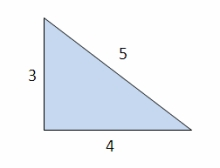# One-quarter 13953

Calculate the magnitude of the interior angles of the triangle ABC if alpha = two-fifths beta and alpha = one-quarter gamma.

A =  24
B =  60
C =  96

### Step-by-step explanation:

A=2/5·B
A = C/4
A+B+C=180

5A-2B = 0
4A-C = 0
A+B+C = 180

Row 2 - 4/5 · Row 1 → Row 2
5A-2B = 0
1.6B-C = 0
A+B+C = 180

Row 3 - 1/5 · Row 1 → Row 3
5A-2B = 0
1.6B-C = 0
1.4B+C = 180

Row 3 - 1.4/1.6 · Row 2 → Row 3
5A-2B = 0
1.6B-C = 0
1.875C = 180

C = 180/1.875 = 96
B = 0+C/1.6 = 0+96/1.6 = 60
A = 0+2B/5 = 0+2 · 60/5 = 24

A = 24
B = 60
C = 96

Our linear equations calculator calculates it.Did you find an error or inaccuracy? Feel free to write us. Thank you!

Tips for related online calculators
Do you have a system of equations and looking for calculator system of linear equations?